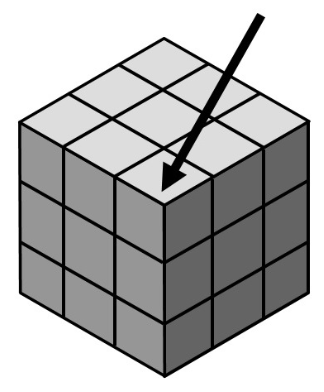### Home > A2C > Chapter 4 > Lesson 4.1.3 > Problem4-45

4-45.

Suppose you have a $3$ by $3$ by $3$ cube. It is painted on all six faces and then cut apart into $27$ pieces, each a $1$ by $1$ by $1$ cube. If one of the cubes is chosen at random, what is the probability that:

1. Three sides are painted?

This cube will have three sides painted. How many cubes like this are there in the figure? How many $1$ by $1$ cubes are there altogether?$P(3\text{ sides painted})=\frac{8}{27}$

1. Two sides are painted?

$P(2\text{ sides painted})=\frac{12}{27}$

1. One side is painted?

How many cubes have one side exposed?

1. No sides are painted?

How many cubes have $1$, $2$, or $3$ sides painted? How many are left?